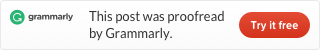Categories

# LeetCode 242 Valid Anagram [sort] java

Source URL: https://leetcode.com/problems/valid-anagram/

Given two strings s and , write a function to determine if t is an anagram of s.

Example 1:

```Input: s = "anagram", t = "nagaram"
Output: true
```

Example 2:

```Input: s = "rat", t = "car"
Output: false
```

Note:
You may assume the string contains only lowercase alphabets.

What if the inputs contain unicode characters? How would you adapt your solution to such case?

This problem we provide three solutions.

## 1. Sort Solution

First, transform two strings into two char arrays, then sort them. Use one loop to compare every element in char arrays. Only all the element is equal the two strings are anagrams to each other. Code:

Running time is 8ms.

## 2. Hashmap solution

We use a hashmap to store the count number of one element in two char arrays. If an element is in array one, we add the count, if an element is in array two, we subtract the count. In the end, if the hashmap is empty, we know they are anagrams. Code:

Running time is 16ms。

## 3. Array Solution

We use a 256 elements array store count information. Code:

Running time is 3 ms faster than 94.23% Java submissions.